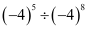# Exponents And Powers Chapter 12 Class 8 Maths NCERT Textbook With Solutions PDF

NCERT Solutions for Class 8 Maths Chapter 12′ PDF Quick download link is given at the bottom of this article. You can see the PDF demo, size of the PDF, page numbers, and direct download Free PDF of ‘Ncert Class 8 Maths Chapter 12 Exercise Solution’ using the download button.

### Chapter 12:Exponents And Powers

#### 12.4 Use of Exponents to Express Small Numbers in Standard Form Observe the following facts.

1. The distance from the Earth to the Sun is 149,600,000,000 m.
2. The speed of light is 300,000,000 m/sec.
3. Thickness of Class VII Mathematics book is 20 mm.
4. The average diameter of a Red Blood Cell is 0.000007 mm.
5. The thickness of human hair is in the range of 0.005 cm to 0.01 cm.
6. The distance of moon from the Earth is 384, 467, 000 m (approx).
7. The size of a plant cell is 0.00001275 m.
8. Average radius of the Sun is 695000 km.
9. Mass of propellant in a space shuttle solid rocket booster is 503600 kg.
10. Thickness of a piece of paper is 0.0016 cm.
11. Diameter of a wire on a computer chip is 0.000003 m.
12. The height of Mount Everest is 8848 m. Observe that there are few numbers which we can read like 2 cm, 8848 m, 6,95,000 km. There are some large numbers like 150,000,000,000 m and some very small numbers like 0.000007 m. Identify very large and very small numbers from the above facts and write them in the adjacent table:
We have learnt how to express very large numbers in standard form in the previous class.

### NCERT Solutions Class 8 Maths Chapter 12 Exponents And Powers

1. Evaluate:

(i) 3-2 (ii) (-4)-2 (iii) (1/2)-5

Solution:

(i) 3-2 = (1/3)2

= 1/9

(ii) (-4)-2 = (1/-4)2

= 1/16

(iii) (1/2)-5 = (2/1)5

= 25

= 32

2.  Simplify and express the result in power notation with positive exponent:

(i) (-4)÷(-4)8

(ii) (1/23)2

(iii) -(3)4×(5/3)4

(iv) (3-7÷3-10)×3-5

(v) 2-3×(-7)-3

Solution:

(i)= (-4)5/(-4)8

= (-4)5-8

= 1/(-4)3

(ii) (1/23)2

= 12/(23)2

= 1/23×2 = 1/26

(iii) -(3)4×(5/3)4

= (-1)4×34×(54/34 )

= 3(4-4)×54

= 30×54 = 54

(iv)

=   (3-7/3-10)× 3-5

= 3-7 – (-10) × 3-5

= 3(-7+10)×3-5

= 33×3-5

= 3(3+-5)

= 3-2

=1/32

(v) 2-3×(-7) – 3

= (2×-7)-3

(Because am×bm = (ab)m)

= 1/(2×-7)3

= 1/(-14)3

NCERT Solutions Class 8 Maths Chapter 12 Exponents And Powers PDF Free Download